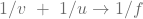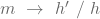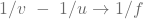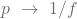February 4, 2022

# Class 10 Science Chapter 10 Light Reflection and Refraction

In the chapter Class 10 Science Chapter 10 Light Reflection and Refraction, we are going to learn many things about light. As you have seen, in our daily life when you turn on the light in a dark room you are able to see the things which are present in the room.

But in the daytime, you are able to see all the things clearly. Ever wonder why is how is this happening. So, this all happens due to light. So, when light falls on an object it throws back the reflection in our eyes, and then we are able to see the object. Light always travels in a straight line.

That light could be coming from the sun or an artificial light source such as light bulbs, tube lights, a torch, etc. Also, all these objects produce white light from it. So, how can we see colors in the object? It’s simple because the light is white it reflects the same color as what object it falls on. Let’s learn more about this.

## Class 10 Science Chapter 10 Light Reflection and Refraction

In this section of Class 10 Science Chapter 10 Light Reflection and Refraction, we are going to learn about the reflection of light and types of mirrors.

# Reflection of Light

Reflection is a phenomenon of light bouncing in the same medium when light falls on it. As you may have already studied in our previous classes, there are 2 laws of reflection.

1. The angle of incidence is equal to the angle of reflection.
2. All 3 rays i.e. incident ray, normal ray, and reflected ray lie in the same place.

There are some features of reflection:

1. When you see yourself in a plane mirror then your image is virtual and erect.
2. Your Image is of the same size as you.
3. Image is inverted, which means your right becomes the image’s left and vice-versa.

# Sperical mirrors

They are of 2 types i.e. concave and convex mirror. Let’s understand both of these in a more clear way.

Concave mirror: This mirror has a reflecting surface that is curved from the inside.

Convex mirror: This mirror has a reflecting surface that is curved from the outside.

Now let’s learn some terminologies regarding this topic:

1. Pole: The center of any mirror known as the pole. It is denoted by “P“.
2. Center of curvature: The curved mirror is a part of a whole circle. So, the center part of that circle is known as the Center of curvature. It is denoted by “C“.
3. The radius of curvature: Distance between from center of curvature to the pole. It is denoted by “R“. In the concave mirror it lies in front of the mirror, but in the convex mirror, it lies behind the mirror.
4.  Principal Axis: It is an imaginary straight line passing through the center of curvature and the pole.
5. Focus or Principal Focus: In the concave mirror it’s a point where all the reflecting rays which are parallel to the principal axis meet together at the same time. While in the convex mirror it is a point from all the rays that appear to come from a point on the principal axis. It is denoted by “F“.
6. Focal Length: It is the distance between pole and focus. It is denoted by “f“.
7. Aperture: The length of a mirror is known as an aperture. On those mirrors that have a smaller aperture, the radius of curvature is double of focal length i.e. R = 2f.

### Class 10 Science Chapter 10 Light Reflection and Refraction

In this section of Class 10 Science Chapter 10 Light Reflection and Refraction, we are going to learn about the uses of spherical mirrors, the formula of mirrors, and magnificant.

# Uses of the concave mirrors

There are many uses of concave mirrors such as. These mirrors help people see an image in a larger size. So, these are used in the Vehicle’s headlights, Shaving mirrors, Solar furnaces, Searchlights, Torches, Flashlights, etc.

# Uses of the convex mirrors

Like the concave mirror, there are also many uses of convex mirrors such as. These mirrors always give a straight, albeit small, image. They have a wide field of view. Thus, these mirrors enable a person to see a much larger area. So, these are mainly used as a rearview mirror in vehicles, vigilance mirror, reflector in street lamps, etc.

# Mirror Formula

The distance of the object from the pole in a spherical mirror is called the distance of the object and is denoted by “u”. The distance of the image from the pole of the mirror is called the distance of the image and is denoted by “v”. The distance from the pole to the principal focus is called the focal length and is denoted by “f”. The relation between these three quantities is known as the mirror formula.

Formula:# Magnificant

It is the process of enlarging the apparent size of an object, not the physical size. This magnification is determined by a calculated number also called “magnification”. When this number is less than one, it indicates a reduction in size. It is represented as a ratio of the height of the image and is denoted by “m”.

h’ → Height of image

h → Height of object

Formula:### Class 10 Science Chapter 10 Light Reflection and Refraction

In this section of Class 10 Science Chapter 10 Light Reflection and Refraction, we are going to learn about the refraction of light.

# Refraction of Light

Refraction is the bending of a wave from one medium to another, the bending of a wave is due to the density of 2 different mediums for example air and glass.

When light travels through a rarer medium (air)  to a denser medium (water) then it bends towards the normal ray. When light travels from the denser (water) to a rarer medium (air) it bends away from the normal ray.

# Refraction through a rectangular glass slab

In this condition, light travels from a rarer medium (air) to a denser medium (glass) and moves towards the normal ray. So, the angle between the incident ray and normal ray is known as the angle of incidence and is denoted by “i”. The angle between normal and refracted ray is known as the angle of refraction and is denoted by “r”.

# Laws of refraction of light

There are 2 laws of refraction of light.

1. The incident ray, the refracted ray, and the normal interface of the two media at the point of incidence all lie on the same plane.
2. The ratio of the sine of the angle of incidence to the sine of the angle of refraction is constant. This law is also known as Snell’s law of refraction.

# Refractive Index

First, you have to memorize that the speed of light is fastest in the air or vacuum i.e. 3×108m/s. Now, let’s understand the refractive index.

The refractive index also called the index of refraction, is a measure of the bending of a beam of light as it passes from one medium to another.

When a light ray travels from medium 1 to medium 2, and if we want to calculate the refractive index value of medium 2 with respect to medium 1 then we need a formula i.e n21 = v1/v2 and vice versa.

### Class 10 Science Chapter 10 Light Reflection and Refraction

In this section of Class 10 Science Chapter 10 Light Reflection and Refraction, we are going to learn about refraction by spherical lenses.

There are 2 types of lenses, one is if a lens has 2 spherical surfaces which are bulging outwards known as a convex lens, and the other is if a lens has 2 spherical surfaces that bend inwards then it is known as a concave lens. The convex lens is also known as the converging lens and the concave lens is also known as a diverging lens.

Now let’s see some terminologies.

1. Center of curvature: The reflecting surface of a spherical mirror forms a part of the sphere. The center of the sphere is called the center of curvature.
2. Principle axis: A line that passes through the center of curvature of a 1 lens to another lens.
3. Optical center: The center part of a lens is known as an optical center and is denoted by “o”.
4. Apparatus: It is known as the diameter or length of the lens.
5. Focus or principle focus: It is a point where a ray of light parallel to the principal axis appears to diverge from a point on the principal axis after passing through the lens.
6. Focal length: It is the distance of the principal focus from the optical center of the lens.

# Sign Convention for Spherical Lenses

Let’s understand this topic by a table.

(*) → special case

Particulars Convex lens sign Concave lens sign
u (object distance) negative (-) negative (-)
v (image distance)* positive (+) and negative (-) negative (-)
f (focal length) positive (+) negative (-)

# Lens formula

u → object distance

v → image distance

f → focal length

Formula:# Magnificant

It is denoted by “m”.

Formula:m > 1 → Enlarged size

m = 1 → Same size

m < 1 → Diminished size

### Class 10 Science Chapter 10 Light Reflection and Refraction

In this section of Class 10 Science Chapter 10 Light Reflection and Refraction, we are going to understand the power of a lens.

The ability of a lens to converge or diverge light rays depends on its focal length which is denoted by “p”. The SI unit of lens power is ‘diopter’. 1 diopter is the power of a lens whose focal length is 1 meter. If the power of a lens is positive then it is a convex lens. Similarly, if the power of a lens is negative then it is a concave lens.

The longer the focal length, the less power the lens has and vice versa. We can also say that the power of a lens is inversely proportional to its focal length.

Formula:Formula:So, this is all the information you want to know about the Class 10 Science Chapter 10 Light Reflection and Refraction. Hopefully, you liked our article on Class 10 Science Chapter 10 Light Reflection and Refraction. If you have any doubts you can ask us in the comment section. We are here to help you out.

Hope we cover every important knowledge of Class 10 Science Chapter 10 Light Reflection and Refraction. If we missed something let us know in the comment section.